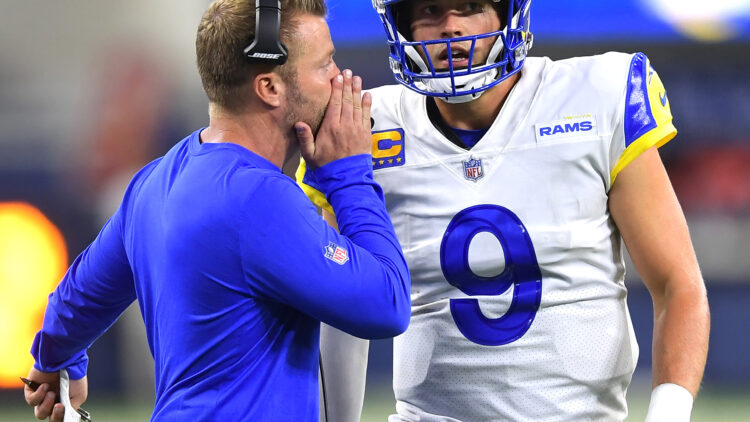# NFL’s Top QBs by PFF, QBR, Passer Rating Formula: After Week 1The following scores are “good” through September 15th, 2021.

There is a healthy fight within the stat community on which quarterback metric is most coherent. Passer rating is bashed as too antiquated. When used to compare quarterbacks from eras of yesteryear, passer rating is indeed skewed and inflated. But touchdowns, passing yards, interception, and completion percentage will never be wholly irrelevant.

ESPN’s QBR is said to factor in more intangible-type ratings. It can be interpreted as a more-detailed and comprehensive version of passer rating and then some.

Pro Football Focus uses its grading system to assess the x’s and o’s of each game and pump out a yearly score.

The following scores and rankings are weighted. Passer rating accounts for 20% of the score, QBR at 40%, and PFF at 40%. Skill position players and players with menial pass attempts were excluded.

# 32. Aaron Rodgers, GB (30.0)

PFF = 43.3, QBR = 13.4, P-Rating = 36.8

# 31. Trevor Lawrence, JAX (42.7)

PFF = 57.7, QBR = 14.2, P-Rating = 70.1

# 30. Ben Roethlisberger, PIT (43.3)

PFF = 38.0, QBR = 28.5, P-Rating = 83.9

# 29. Matt Ryan, ATL (45.5)

PFF = 60.4, QBR = 17.6, P-Rating = 71.63

# 28. Ryan Tannehill, TEN (47.7)

PFF = 64.3, QBR = 17.5, P-Rating = 74.9

# 27. Zach Wilson, NYJ (53.3)

PFF = 63.2, QBR = 28.7, P-Rating = 82.9

# 26. Andy Dalton, CHI (53.7)

PFF = 63.1, QBR = 34.9, P-Rating = 72.9

# t25. Tua Tagovailoa, MIA (54.9)

PFF = 57.8, QBR = 39.8, P-Rating = 79.6

# t25. Carson Wentz, IND (54.9)

PFF = 60.1, QBR = 26.3, P-Rating = 102.0

# 23. Jared Goff, DET (55.1)

PFF = 66.7, QBR = 24.8, P-Rating = 92.6

# 22. Taylor Heinicke, WAS (57.8)

PFF = 46.9, QBR = 38.1, P-Rating = 119.3

# 21. Josh Allen, BUF (59.2)

PFF = 67.6, QBR = 40.7, P-Rating = 79.7

# 20. Daniel Jones, NYG (63.7)

PFF = 65.1, QBR = 49.0, P-Rating = 90.7

# 19. Sam Darnold, CAR (67.2)

PFF = 70.1, QBR = 47.1, P-Rating = 102.0

# 18. Lamar Jackson, BAL (68.0)

PFF = 63.1, QBR = 57.7, P-Rating = 98.6

# 17. Derek Carr, LV (68.9)

PFF = 74.9, QBR = 52.8, P-Rating = 89.5

# 16. Kirk Cousins, MIN (72.4)

PFF = 80.9, QBR = 46.7, P-Rating = 106.8

# 15. Justin Herbert, LAC (75.3)

PFF = 84.7, QBR = 61.1, P-Rating = 85.2

# 14. Baker Mayfield, CLE (76.8)

PFF = 75.1, QBR = 68.3, P-Rating = 97.5

# 13. Joe Burrow, CIN (77.7)

PFF = 69.7, QBR = 60.2, P-Rating = 128.8

# 12. Dak Prescott, DAL (81.0)

PFF = 77.6, QBR = 74.3, P-Rating = 101.4

# 11. Tyrod Taylor, HOU (81.3)

PFF = 71.1, QBR = 76.1, P-Rating = 112.1

# 10. Mac Jones, NE (81.8)

PFF = 78.3, QBR = 75.1, P-Rating = 102.6

# 9. Tom Brady, TB (82.7)

PFF = 93.0, QBR = 65.3, P-Rating = 97.0

# 8. Jimmy Garoppolo, SF (83.1)

PFF = 71.2, QBR = 74.6, P-Rating = 124.2

# 7. Kyler Murray, ARI (89.8)

PFF = 84.5, QBR = 79.5, P-Rating = 121.0

# 6. Jalen Hurts, PHI (90.2)

PFF = 89.5, QBR = 72.8, P-Rating = 126.4

# 5. Patrick Mahomes, KC (93.8)

PFF = 81.0, QBR = 88.0, P-Rating = 131.4

# 4. Teddy Bridgewater, DEN (95.7)

PFF = 85.7, QBR = 95.7, P-Rating = 115.7

# 3. Russell Wilson, SEA (95.9)

PFF = 79.1, QBR = 84.6, P-Rating = 152.3

# 2. Jameis Winston, NO (98.9)

PFF = 89.5, QBR = 92.5, P-Rating = 130.8

# 1. Matthew Stafford, LAR (99.0)

PFF = 79.7, QBR = 89.9, P-Rating = 156.1
Dustin Baker is a political scientist who graduated from the University of Minnesota in 2007. He hosts a podcast with Bryant McKinnie, which airs every Wednesday with Raun Sawh and Sally from Minneapolis. His Viking fandom dates back to 1996. Listed guilty pleasures: Peanut Butter Ice Cream, ‘The Sopranos,’ and The Doors (the band).

Share: